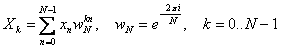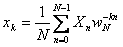# Fast Fourier transform

Discrete Fourier transform transforms a sequence of complex or real numbers x into a sequence of complex numbers X. Forward and inverse Fourier transforms are defined as follows:The formulas above have the O(N 2) complexity. However, there is a well-known way of decreasing the complexity of discrete Fourier transform to O(N·log(N)). Fast Fourier transform is widely used as such and also to speed up calculation of other transforms - convolution and cross-correlation.

# Implementation of FFT in ALGLIB

ALGLIB package supports fast Fourier transforms of complex sequences of any length. In case the length of transform N is a composite number, Cooley-Tukey algorithm is used, which converts the initial Fourier transform to shorter transforms that correspond to prime factors of N. Short length transforms (N ≤ 5) are calculated using special formulas for short transforms; longer transforms that correspond to prime N or large prime factors of composite N, are calculated using Bluestein algorithm. As a result, the algorithm has the speed of O(N·log(N)) for any N - prime or composite. However, some transforms are calculated faster than the others. If the transform length is a prime number or a composite number with large prime factors, the transform may require 4-6 times more time than if the length is a closely adjacent composite number with small prime factors.

# Real FFT

Most FFT algorithms were developed for complex sequences, as complex case can be analyzed more easily than real. However, in practice one often has to work with real numbers and thus the speed of real FFT is a separate issue. For even N there is a formula that allows to convert a real FFT to a complex FFT that is half the length and thus increase the speed twice as compared to the complex fast FT of the same length. However, for odd N there is no simple way of increasing the transform speed and this problem has not yet been solved in ALGLIB.

Thus, if the length of the transform is an even number, the real transform will be approximately twice faster than the complex transform. If the length of the transform is an odd number, the speed of real transform will be the same as the speed of complex transform of the same length.

ALGLIB Project offers you two editions of ALGLIB:

ALGLIB Free Edition:delivered for freeoffers full set of numerical functionalityextensive algorithmic optimizationsno low level optimizationsnon-commercial license

ALGLIB Commercial Edition:flexible pricingoffers full set of numerical functionalityextensive algorithmic optimizationshigh performance (SMP, SIMD)commercial license with support plan

## ALGLIB 3.17.0 for C++C++ library.
Delivered with sources.
Monolithic design.
Extreme portability.
Editions:   FREE   COMMERCIAL

## ALGLIB 3.17.0 for C#C# library with native kernels.
Delivered with sources.
VB.NET and IronPython wrappers.
Extreme portability.
Editions:   FREE   COMMERCIAL

## ALGLIB 3.17.0 for DelphiDelphi wrapper around C core.
Delivered as precompiled binary.
Compatible with FreePascal.
Editions:   FREE   COMMERCIAL

## ALGLIB 3.17.0 for CPythonCPython wrapper around C core.
Delivered as precompiled binary.
Editions:   FREE   COMMERCIAL